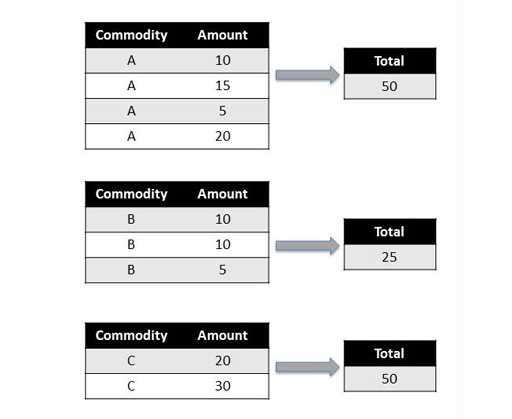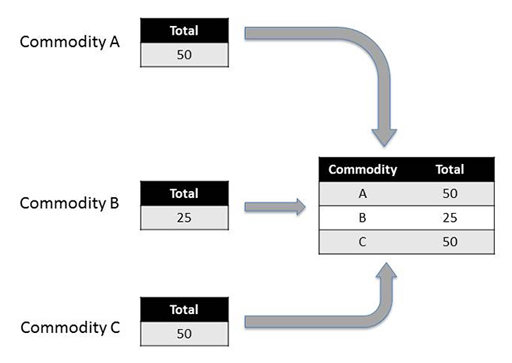Digital & Computing

### Become an OU studentLearn to code for data analysis

Start this free course now. Just create an account and sign in. Enrol and complete the course for a free statement of participation or digital badge if available.

# 1.3 Summary operations

Summary, or aggregation, operations are used to produce a single summary value or statistic, such as the group average, for each separate group.

Find the ‘total’ amount within each group using a summary operation:Figure 4

To apply a summary operator to each group, such as a function to find the mean value of each group, and then automatically combine the results into a single output dataframe, pass the name of the function in to the aggregate() method. Note that pandas will try to use this operator to summarise each column in the grouped rows separately if there is more than one column that can be summarised. So for example, if there was a ‘Volume’ column, it would also return total volumes.

Let’s use again the example dataframe defined earlier:

In []:

df

Out[]:

CommodityAmount
0A10
1A15
2A5
3A20
4B10
5B10
6B5
7C20
8C30

Group the data by commodity type and then apply the sum operation and combine the results in an output dataframe. The grouping elements are used to create index values in the output dataframe.

In []:

grouped=df.groupby('Commodity')

grouped.aggregate(sum)

Out[]:

Amount
Commodity
A50
B25
C50

In this case, the aggregate() method applies the sum summary operation to each group and then automatically combines the results. For a summary operation such as this, the resulting combined dataframe contains as many rows as there were groups created by the splitting .groupby() operation.Figure 5

The slightly more general apply() method can also be substituted for the aggregate() method and will similarly take the rows associated with each group, apply a function to them, and return a combined result.

The apply() method can be really handy if you have defined a function of your own that you want to apply to just the rows associated with each group. Simply pass the name of the function to the apply() method and it will then call your function, once per group, on the sets of rows associated with each group.

For example, find the top two items by ‘Amount’ in each group:

In []:

def top2byAmount(g):

grouped.apply(top2byAmount)

Out[]:

Amount
Commodity
A320
115
B410
510
C830
720

The second index column containing the numbers 3, 1, 4 etc., contains the original index value of each row.

In Week 3 the apply() method was called on a column, to apply the given function to each cell. Here it was called on a grouped dataframe, to apply the given function to each group.

## Exercise 3 Experimenting with split-apply-combine

Work through Exercise 3 in your Exercise notebook 4 to practise the summary operations.